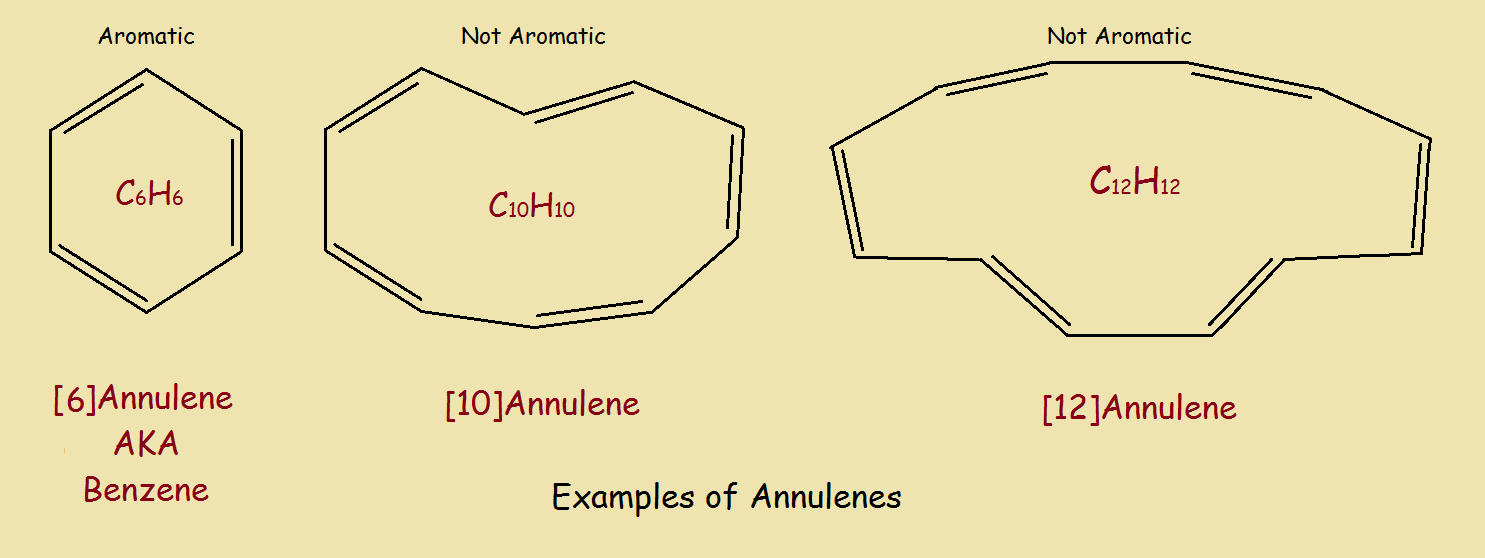# Annulene and Two Simple Derivatives – Aromaticity?

1. Home
2. /
3. Chemistry
4. /
5. Annulene and Two Simple...Complex chemicals possess names almost equally complex. Occasionally, a simpler nomenclature can be employed. For basic aromatic compounds, composed of a ring of alternating single and double bonded carbon atoms in a ring, the name [n]annulene has been is commonly used. Examples, including annulene are given below.## Aromaticity

We won’t go into the theory behind aromaticity. What we will do is lay out the basic factors that students use to determine if a compound is aromatic.

1. The ring is composed of conjugated single and double bonds (…−C=C−C=…).
2. The molecule is relatively flat.
3. The number of available π-electrons equals 4n + 2 (a Hückel number), where n is
generally a small positive integer.¹
4. Crowding does not severely limit or prevent aromaticity.
5. Ring size affects aromaticity, but does not automatically prohibit it (see ref. 1).

For instance, the benzene molecule is composed of 6 conjugated single and double bonds. It is flat. And the number of π-electrons is 2 from each double bond, giving a total of 6 – a good number, since 4(1) + 2 = 6.

This compound crystallizes out as dark, brick-red crystals that are air-sensitive. It decomposes at 96°C. Due to the triple bonds, it possesses 18 π-electrons. Six of these, however, do not contribute to any potential aromaticity, since their orbitals are orthogonal² to those of the other π-electrons.

Contributing π-electrons amount to 12 in number. This is not a Hückel number, however, since 12 = 4n with n=3. Since it is not 4n + 2, the molecule is not aromatic. If the 6 other π-electrons did add in, the molecule would have been aromatic with n=4!

## Annulene Derivatives: Answering Our Initial Inquiry

While we do not have test results to prove our two compounds illustrated in Figure 1 are aromatic, they seem to meet the following criteria:1. Both molecules consist completely of conjugated double bonds, even if
more than one ring.
2. The two molecules are flat.
3. Both molecules possess the required 4n + 2 number of π-electrons.
4. Crowding does not appear to be a problem.
5. Ring size falls well within reasonable range.

We thus state, until proven otherwise, that the two annulene derivatives we introduced in our discussion are, indeed, aromatic organic compounds.

¹ Erich Hückel developed this equation in connection with molecular orbital theory.
² Orthogonality is a mathematics/physics concept describing how two properties act
independently, in effect, “perpendicular” to each other, “ignoring” each other.

Note: You might also enjoy Is Cyclopropenone Aromatic or Not?

References: